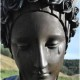## Fibering the figure-8 knot complement over the circle

October 11, 2010

As I was making some false statements about how I think geometrically finite ends of a hyperbolic three manifold would look like, professor Gabai pointed out this super cool fact (proved by Cannon and Thurston, 2007) that the figure-eight knot complement admits a hyperbolic structure and fibers over the circle, but if we lift any fiber (which would be a surface) into the hyperbolic 3-space, the resulting surface would be an embedded topological disc with limit set being the whole limit 2-sphere (!) i.e. if we see$\mathbb{H}^3$ as a Euclidean open ball, then the boundary of such a disc is a Peano curve that covers the whole 2-sphere bounding$\mathbb{H}^3$.

I have read about the hyperbolic structure on the figure-8 knot complement in Thurston’s notes (4.3) (A similar construction can be found in my pervious post about hyperbolic structure on the Whitehead link complement), but I didn’t know the fibering over circle part, so I decided to figure out what this fibration would look like.

After playing with chicken wire and playdo for a few days, I am finally able to visualize the fibration. Here I want to point out a few simple points discovered in the process.

Start with the classical position of the figure-8 knot (two ends extends to infinity and meet at the point infinity in$\mathbb{S}^3$):To find a fibration over the circle, we need to give a surface that spans the knot (such surface is called a Seifert surface) and a homotopy of the surface$\varphi: S \times [0,1] \rightarrow \mathbb{S}^3 \backslash K$ which restricts to a bijection from$S \times [0,1)$ to$\mathbb{S}^3 \backslash K$ and$\varphi(S\times\{1\}) = \varphi(S\times\{0\})$.

For quite some time, I tried with the following surface:Since it’s perfectly symmetric (via a rotation by$\pi$), we only need to produce a homotopy that sends$S$ to the symmetric Seifert surface in the upper half plane. I was not able to find one. (I’m still curious if there is such homotopy, if so, then there are more than one way the knot complement can fiber)

It turns out that there are in fact non-homeomorphic (hence of course non-homotopic) Seifert surfaces spanning the knot, the one I end up using for the fibration is the following surface:Or equivlently, we may connect the two ends at a finite point.To see the boundary is indeed the figure-8 knot:Note that this surface is not homeomorphic to the pervious one because this one is orientable and the pervious is not.

Now I’ll leave it as a brain exercise to see the homotopy. (well…this is largely because it takes forever to draw enough pictures for expressing that) A hint on how the it goes: think of the homotopy as a continuous family of disjoint Seifert surfaces that ‘swipes through’ the whole$\mathbb{S}^3 \backslash K$ and returns to the initial one. As in the picture above, our surface is like a disc with two intertwined stripe handles on it, each handle is two twists in it. The major step is to see that one can ‘pass’ the disc through a double-twisted handle by making the interior of the old disc to become the interior of the new handle. i.e. we can homotope the bowl from under the strap to above the strap with a family of disjoint surfaces with same boundary.In in figure-8 knot case, the disc would need to pass through both straps and return to itself.

### 5 Responses to “Fibering the figure-8 knot complement over the circle”

1.Traintrucks « Area 777 Says:

[…] Fibering the figure-8 knot complement over the circle […]

2.Joe Quinn Says:

Hi, I noticed this is a couple years old but thought I’d try asking this anyway. A book I’m reading mentions that the figure 8 knot complement fibers over the circle with fiber a punctured torus, yet I can’t find any details on this in my other books or online. Do you happen to know if the surface you’ve described here is homotopic to a punctured torus? I’ve been thinking of it like a disk with a handle, but it’s still not obvious. I like what you said about chicken wire and play dough – I seriously think I’m going to try that. But if you happen to know anything about how to get a punctured torus as a fiber, it could save me some precious time. Thanks for your nice drawings either way!

3.Saul Schleimer Says:

The first surface you drew is non-orientable. So it cannot be the fiber of a surface bundle structure on an orientable three-manifold. (Seifert surfaces are usually assumed to be orientable, I think.)

It follows by considering the second (relative) homology group that knot complements fiber in exactly one way. This isn’t true of link complements: even the Hopf link fibers in infinitely many ways. You might think about finding fibrations of the Whitehead link – drawings or, better, animations of the fiberings would be fun to see. For more in this line, you’ll have to read Thurston’s paper on the Thurston norm ball.

4.mrskrishan Says:

neat soap-film (transparent CAD drawing of a torus not bounding a solid torus) Mathematic visualization http://mathematica.stackexchange.com/questions/16638/drawing-a-torus-not-bounding-a-solid-torus

walk through 3-D manifold elaboration “Triangulating the figure 8 knot complement ” https://www.youtube.com/watch?v=k6QNuuK9Ae4

3-D printed solid http://www.shapeways.com/product/C27TWPDU2/figure-8-knot-complement

5.Fun drawing and fibred knots | Serious Maths and Unicorns Says:

[…] you like thinking about these pictures, I would really like to draw your attention to this post by conan777 about fibering of the figure eight knot. Personally I haven’t quite got my head around all of it, but maybe you […]